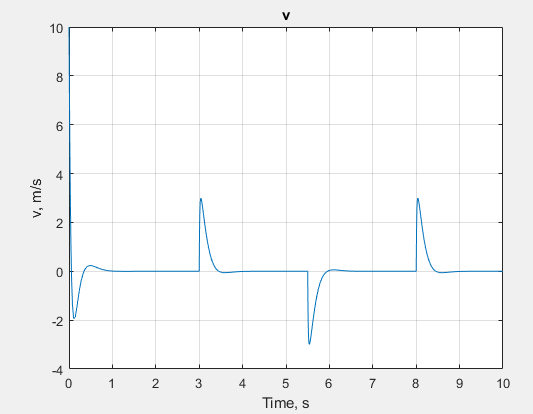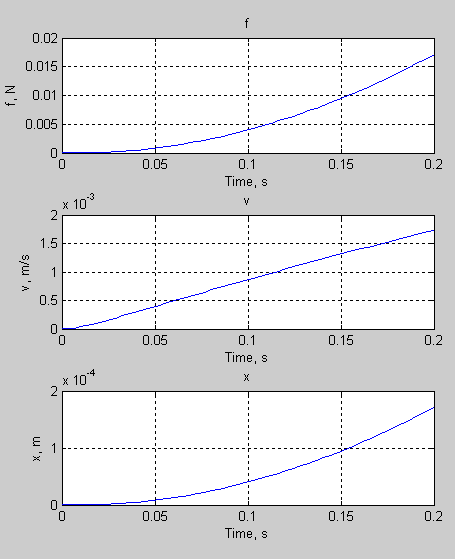# plot

Plot all series associated with Node object

## Syntax

``fh = plot(node)``
``fh = plot(node,Name,Value)``

## Description

example

````fh = plot(node)` plots all nonempty series associated with the specified node and its children. `fh` is a structure of handles to the resulting figures. `node` is a `simscape.logging.Node` object. `node` must include a full identifier path to the node, starting with the workspace log variable name.```

example

````fh = plot(node,Name,Value)` lets you filter the data being plotted by using one or more `Name,Value` pair arguments. For example, specify '`units`' followed by a unit name to plot only nodes that are commensurate with the specified unit.```

## Examples

collapse all

Plot velocity of port R of a Translational Spring block.

Open the Mass-Spring-Damper with Controller example model:

`ssc_mass_spring_damper_control`

This example model has data logging enabled for the whole model, with the Workspace variable name parameter set to `simlog_ssc_mass_spring_damper_control`.

Simulate the model to log the simulation data:

`sim('ssc_mass_spring_damper_control');`

Plot velocity of port R of the Translational Spring block `Spring`.

`plot(simlog_ssc_mass_spring_damper_control.Spring.R);`This command plots all the variables associated with a Translational Spring block, but not with its ports. The command assumes that he model has the default workspace variable name, `simlog`, and that the Translational Spring block is located at the top level of the model diagram.

```plot(simlog.Translational_Spring, 'depth', 1) ```## Input Arguments

collapse all

Simulation data to plot, specified as a `simscape.logging.Node` object or a homogeneous cell array of such objects. `node` must include a full identifier path to the node, starting with the workspace log variable name.

The table describes the resulting plots based on the type of the `node` argument:

 `Node` object Plots all nonempty series associated with the `Node` and its children (up to the level defined by the `depth`). If the `Node` has multiple simulation variable nodes as children at level 1, these children are plotted in the same figure window but on a different axis. Descendants at other levels are plotted in different figure windows. All dimensions of a nonscalar series are plotted on the same axis. Cell array of `Node` objects Plots commensurate series superimposed on the same axis.Intended for use to compare simulation data from different runs. All entries of the cell array are required to be equivalent to each other, meaning that the `Node` objects must have same hierarchy, and the series for each node must have the same dimensions and commensurate units.

### Name-Value Arguments

Specify optional pairs of arguments as `Name1=Value1,...,NameN=ValueN`, where `Name` is the argument name and `Value` is the corresponding value. Name-value arguments must appear after other arguments, but the order of the pairs does not matter.

Before R2021a, use commas to separate each name and value, and enclose `Name` in quotes.

Example: `fh = plot(simlog,'units','mm','time',[1 3])` plots all of the linear position variables in the model (series that are commensurate with units of `mm`), in those units, within the time range between 1 and 3 seconds.

Number of children levels to plot, specified as the comma-separated pair consisting of '`depth`' and a nonnegative integer. By default, the function plots all descendants of `node` that have nonempty series. Specifying `depth` lets you limit the number of levels to plot, for example:

 `'depth',0` No children; plot the nonempty series of the specified node only. `'depth',1` Plot the nonempty series of the specified node and its children. `'depth',2` Plot the nonempty series of the specified node, its children, and their children.

Plot legend, specified as the comma-separated pair consisting of '`names`' and a cell array of character vectors or string scalars. The number of elements in the cell array must be same as the number of elements of `node`.

By default, plots have no legend.

Time range for plotting the data, specified as the comma-separated pair consisting of '`time`' and a 1x2 vector, ```[start_time end_time]```, in seconds.

`[]` plots all data.

Units for plotting the data, specified as the comma-separated pair consisting of '`units`' and a unit name, or a cell array of unit names. Unit names must appear inside single quotes (`''`) or double quotes (`""`).

This argument plots the series values in the specified units, and also filters the data to plot only nodes that have series commensurate with the specified unit.

Example: `fh = plot(simlog,'units',{'mm','mm/s'})` plots all of the linear position and velocity variables in the model (series that are commensurate with units of `mm` and `mm/s`), in those units.

Alternative destination to plot the data, specified as the comma-separated pair consisting of '`viewer`' and `datainspector` inside single quotes (`''`) or double quotes (`""`).

If you specify this name-value pair argument, the function plots the data in the Simulation Data Inspector. By default, the function plots the data in a plot window.

## Output Arguments

collapse all

Handles to the resulting plot figure windows, returned as a structure with the same hierarchy as the `node` being plotted. For example, if a specific child is not plotted then that field in the output structure is empty.

## Version History

Introduced in R2010b